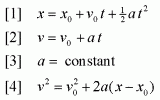# Question regarding Motion in two or three dimensions.

• nyguy

## Homework Statement

Two crickets, Chirpy and Milada, jump from the top of a vertical cliff. Chirpy jumps horizontally and reaches the ground in 3.50s. Milada jumps with an initial velocity of 95.0 cm/s at an angle of 32.0 degrees above the horizontal. How far from the base of the cliff will Milada hit the ground.

## Homework Equations

The equations below SHOULD meet the requirements to finding the answer to this problem.## The Attempt at a Solution

Initially when I approached this problem, it made sense to find the height of the cliff. So, I took the first cricket's time (Chirpy) which was 3.50s and used it to find the height. I used Equation 1. The height that I came up with was 60.025m. In most cases, if not all, the units when dealing with velocity and acceleration problems are usually in meters per second. So, I converted the velocity vector to meters/second and then attempted at finding its corresponding components. After the conversion, 95.0 cm/s is equivalent to .95 m/s. Since this problem is in 2-dimension, I just had to find Vx and Vy, the components of the velocity vector.

The components are as follows:

Vx=(Cos 32) x .95 = .806 m/s

Vy= (Sin 32) x .95 = .503 m/s

Assuming that I'm making the right decision, I made an attempt at finding the second cricket's (Milada) max height when it jumped off of the cliff at a 32 degree angle above the horizontal. I used Equation 4. I have an understanding that when a particle, projectile, or living thing reaches a max height in the air, the velocity is zero due to the constant acceleration pushing down on it. So, I set equation 4 to zero.

These were my calculations:

0=-(.503)^2 + 2(-9.8)(y)
y=.013m

The last thing I did before I was lost was added the max height onto the cliff's height which was 60.038m. I didn't have a clue as to what to do next. How do I find how far from the base of the cliff the second cricket will land?? Do I need to find a new time, a new velocity since the cricket is now in free fall, what?? Please help.